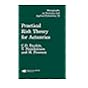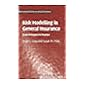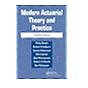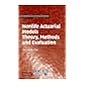Normal view

# Risk modelling in general insurance : from principles to practice / Roger J. Gray, Susan M. Pitts.

Material type:TextLanguage: English Publication details: 2012. Description: xiv, 393 p. : ill. ; 24 cmISBN: 9780521863940 (hardback); 9781139516556; 1139516558DDC classification: 368.01 LOC classification: HG8054.5 | .G735 2012Other classification: MAT003000 Online resources: Worldcat details
Contents:
TOC Cover; Risk Modelling in General Insurance; Series Page; Title; Copyright; Contents; Preface; 1: Introduction; 1.1 The aim of this book; 1.2 Notation and prerequisites; 1.2.1 Probability; 1.2.2 Statistics; 1.2.3 Simulation; 1.2.4 The statistical software package R; 2: Models for claim numbers and claim sizes; 2.1 Distributions for claim numbers; 2.1.1 Poisson distribution; 2.1.2 Negative binomial distribution; 2.1.3 Geometric distribution; 2.1.4 Binomial distribution; 2.1.5 A summary note on R; 2.2 Distributions for claim sizes; 2.2.1 A further summary note on R. 2.2.2 Normal (Gaussian) distribution2.2.3 Exponential distribution; 2.2.4 Gamma distribution; 2.2.5 Fat-tailed distributions; 2.2.6 Lognormal distribution; 2.2.7 Pareto distribution; 2.2.8 Weibull distribution; 2.2.9 Burr distribution; 2.2.10 Loggamma distribution; 2.3 Mixture distributions; 2.4 Fitting models to claim-number and claim-size data; 2.4.1 Fitting models to claim numbers; 2.4.2 Fitting models to claim sizes; Exercises; 3: Short term risk models; 3.1 The mean and variance of a compound distribution; 3.2 The distribution of a random sum. 3.2.1 Convolution series formula for a compound distribution3.2.2 Moment generating function of a compound distribution; 3.3 Finite mixture distributions; 3.4 Special compound distributions; 3.4.1 Compound Poisson distributions; 3.4.2 Compound mixed Poisson distributions; 3.4.3 Compound negative binomial distributions; 3.4.4 Compound binomial distributions; 3.5 Numerical methods for compound distributions; 3.5.1 Panjer recursion algorithm; 3.5.2 The fast Fourier transform algorithm; 3.6 Approximations for compound distributions; 3.6.1 Approximations based on a few moments. 3.6.2 Asymptotic approximations3.7 Statistics for compound distributions; 3.8 The individual risk model; 3.8.1 The mean and variance for the individual risk model; 3.8.2 The distribution function and moment generating function for the individual risk model; 3.8.3 Approximations for the individual risk model; Exercises; 4: Model based pricing -- setting premiums; 4.1 Premium calculation principles; 4.1.1 The expected value principle (EVP); 4.1.2 The standard deviation principle (SDP); 4.1.3 The variance principle (VP); 4.1.4 The quantile principle (QP); 4.1.5 The zero utility principle (ZUP). 4.1.6 The exponential premium principle (EPP)4.1.7 Some desirable properties of premium calculation principles; 4.1.8 Other premium calculation principles; 4.2 Maximum and minimum premiums; 4.3 Introduction to credibility theory; 4.4 Bayesian estimation; 4.4.1 The posterior distribution; 4.4.2 The wider context of decision theory; 4.4.3 The binomial/beta model; 4.4.4 The Poisson/gamma model; 4.4.5 The normal/normal model; 4.5 Bayesian credibility theory; 4.5.1 Bayesian credibility estimates under the Poisson/gamma model; 4.5.2 Bayesian credibility premiums under the normal/normal model.
Summary: A wide range of topics to give students a firm foundation in statistical and actuarial concepts and their applications.
List(s) this item appears in: Management
Tags from this library: No tags from this library for this title.
Star ratingsAverage rating: 0.0 (0 votes)
Holdings
Item type Current library Collection Call number Copy number Status Date due Barcode Item holdsText
Reserve Section
Non-fiction 368.01 GRR 2012 (Browse shelf(Opens below)) C-1 Not For Loan 27385Text
Circulation Section
Non-fiction 368.01 GRR 2012 (Browse shelf(Opens below)) C-2 Available 28165
Total holds: 0
##### Browsing EWU Library shelves, Shelving location: Circulation Section Close shelf browser (Hides shelf browser)368.01 ACT 1997 Actuarial mathematics / 368.01 DAP 1994 Practical risk theory for actuaries / 368.01 DIA 2013 Actuarial mathematics for life contingent risks / 368.01 GRR 2012 Risk modelling in general insurance : 368.01 MOD 2005 Modern actuarial theory and practice / 368.01 PEF 2015 Fundamentals of actuarial mathematics / 368.01 TSN 2009 Nonlife actuarial models :

4.6 Empirical Bayesian credibility theory: Model 1 - the Bühlmann model.

Includes bibliographical references (p. 386-388) and index.

TOC Cover; Risk Modelling in General Insurance; Series Page; Title; Copyright; Contents; Preface; 1: Introduction; 1.1 The aim of this book; 1.2 Notation and prerequisites; 1.2.1 Probability; 1.2.2 Statistics; 1.2.3 Simulation; 1.2.4 The statistical software package R; 2: Models for claim numbers and claim sizes; 2.1 Distributions for claim numbers; 2.1.1 Poisson distribution; 2.1.2 Negative binomial distribution; 2.1.3 Geometric distribution; 2.1.4 Binomial distribution; 2.1.5 A summary note on R; 2.2 Distributions for claim sizes; 2.2.1 A further summary note on R. 2.2.2 Normal (Gaussian) distribution2.2.3 Exponential distribution; 2.2.4 Gamma distribution; 2.2.5 Fat-tailed distributions; 2.2.6 Lognormal distribution; 2.2.7 Pareto distribution; 2.2.8 Weibull distribution; 2.2.9 Burr distribution; 2.2.10 Loggamma distribution; 2.3 Mixture distributions; 2.4 Fitting models to claim-number and claim-size data; 2.4.1 Fitting models to claim numbers; 2.4.2 Fitting models to claim sizes; Exercises; 3: Short term risk models; 3.1 The mean and variance of a compound distribution; 3.2 The distribution of a random sum. 3.2.1 Convolution series formula for a compound distribution3.2.2 Moment generating function of a compound distribution; 3.3 Finite mixture distributions; 3.4 Special compound distributions; 3.4.1 Compound Poisson distributions; 3.4.2 Compound mixed Poisson distributions; 3.4.3 Compound negative binomial distributions; 3.4.4 Compound binomial distributions; 3.5 Numerical methods for compound distributions; 3.5.1 Panjer recursion algorithm; 3.5.2 The fast Fourier transform algorithm; 3.6 Approximations for compound distributions; 3.6.1 Approximations based on a few moments. 3.6.2 Asymptotic approximations3.7 Statistics for compound distributions; 3.8 The individual risk model; 3.8.1 The mean and variance for the individual risk model; 3.8.2 The distribution function and moment generating function for the individual risk model; 3.8.3 Approximations for the individual risk model; Exercises; 4: Model based pricing --
setting premiums; 4.1 Premium calculation principles; 4.1.1 The expected value principle (EVP); 4.1.2 The standard deviation principle (SDP); 4.1.3 The variance principle (VP); 4.1.4 The quantile principle (QP); 4.1.5 The zero utility principle (ZUP). 4.1.6 The exponential premium principle (EPP)4.1.7 Some desirable properties of premium calculation principles; 4.1.8 Other premium calculation principles; 4.2 Maximum and minimum premiums; 4.3 Introduction to credibility theory; 4.4 Bayesian estimation; 4.4.1 The posterior distribution; 4.4.2 The wider context of decision theory; 4.4.3 The binomial/beta model; 4.4.4 The Poisson/gamma model; 4.4.5 The normal/normal model; 4.5 Bayesian credibility theory; 4.5.1 Bayesian credibility estimates under the Poisson/gamma model; 4.5.2 Bayesian credibility premiums under the normal/normal model.

A wide range of topics to give students a firm foundation in statistical and actuarial concepts and their applications.

AS

Sagar Shahanawaz

There are no comments on this title.